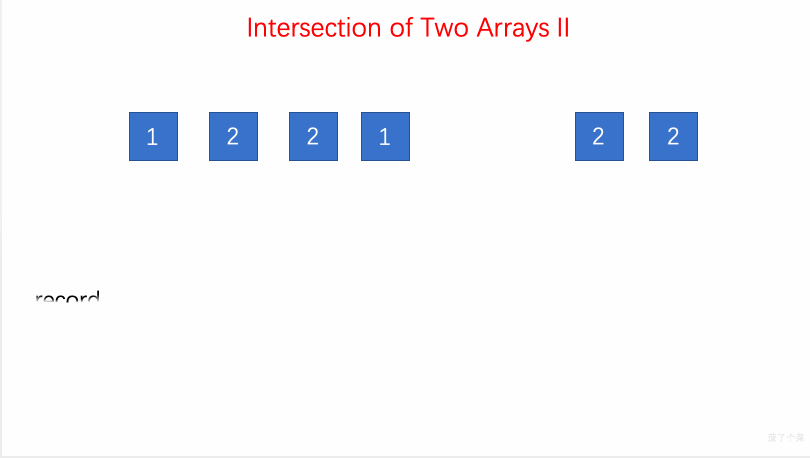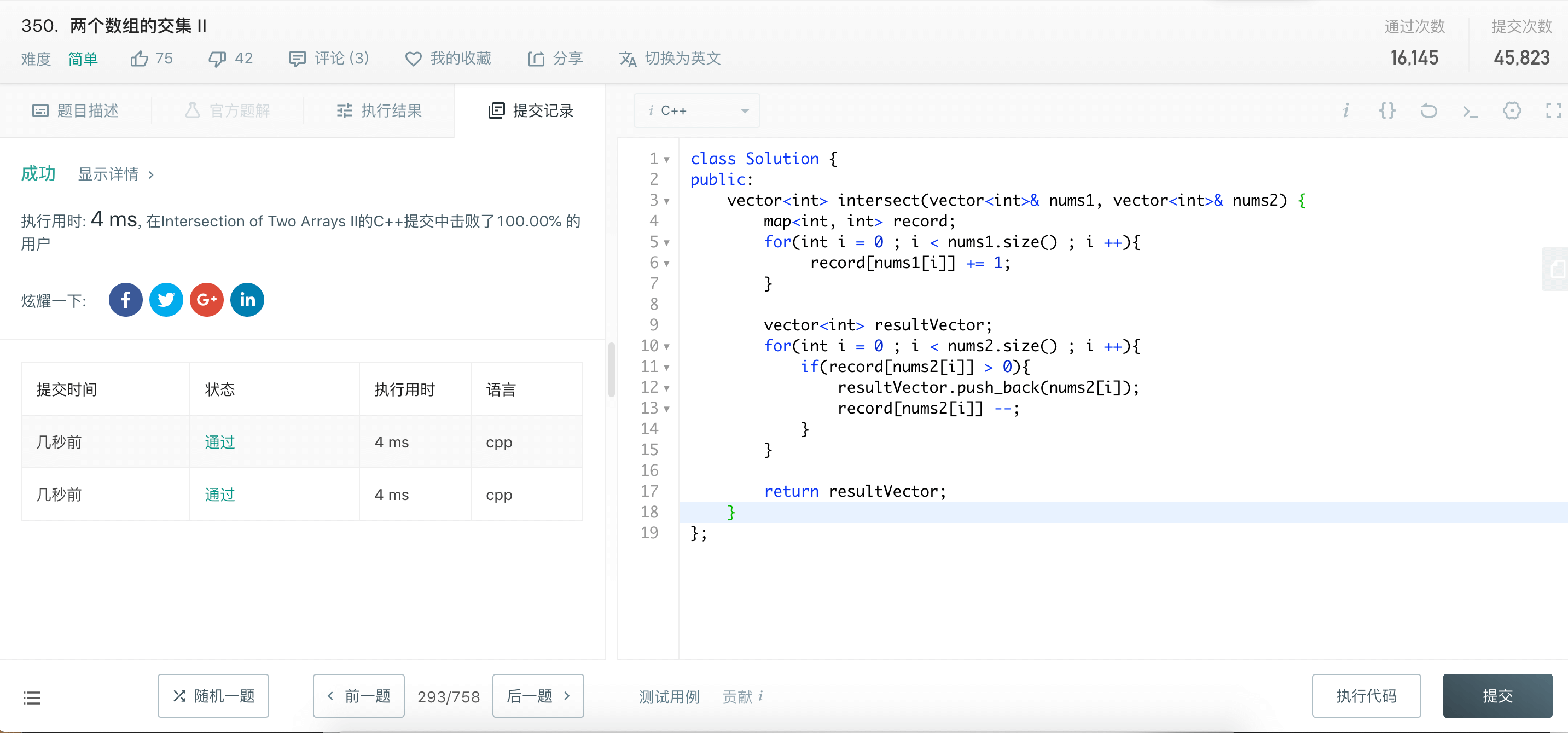# LeetCode 第 350 号问题：两个数组的交集 II

### 题目描述

``````输入: nums1 = [1,2,2,1], nums2 = [2,2]

``````

``````输入: nums1 = [4,9,5], nums2 = [9,4,9,8,4]

``````

• 输出结果中每个元素出现的次数，应与元素在两个数组中出现的次数一致。
• 我们可以不考虑输出结果的顺序。

• 如果给定的数组已经排好序呢？你将如何优化你的算法？
• 如果 nums1 的大小比 nums2 小很多，哪种方法更优？
• 如果 nums2 的元素存储在磁盘上，磁盘内存是有限的，并且你不能一次加载所有的元素到内存中，你该怎么办？

### 题目解析

• 遍历 num1，通过map容器 record 存储 num1 的元素与频率
• 遍历 num2 ，在 record 中查找是否有相同的元素（该元素的存储频率大于0），如果有，用map容器resultVector 进行存储，同时该元素的频率减一

### 动画描述### 代码实现

``````// 350. Intersection of Two Arrays II
// https://leetcode.com/problems/intersection-of-two-arrays-ii/description/
// 时间复杂度: O(nlogn)
// 空间复杂度: O(n)
class Solution {
public:
vector<int> intersect(vector<int>& nums1, vector<int>& nums2) {

map<int, int> record;
for(int i = 0 ; i < nums1.size() ; i ++){
record[nums1[i]] += 1;
}

vector<int> resultVector;
for(int i = 0 ; i < nums2.size() ; i ++){
if(record[nums2[i]] > 0){
resultVector.push_back(nums2[i]);
record[nums2[i]] --;
}
}

return resultVector;
}
};
``````

#### 执行结果